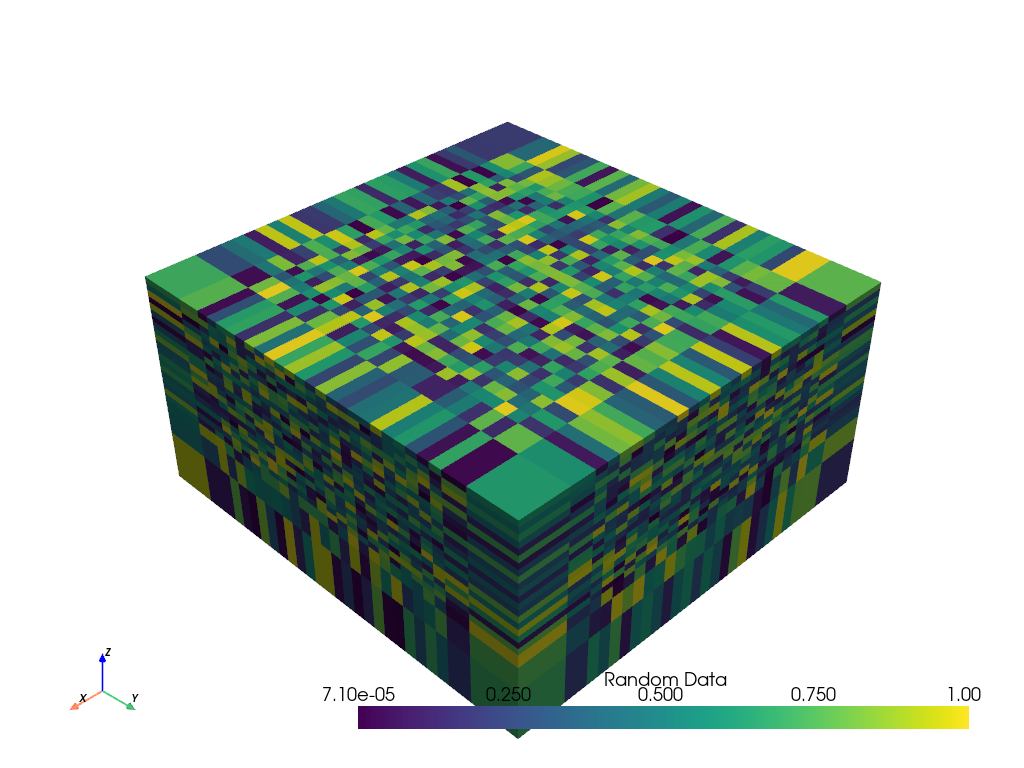# Append Cell Centers¶

This example will demonstrate how to append a dataset’s cell centers as a length 3 tuple array.

This example demonstrates `PVGeo.filters.AppendCellCenters`

```from pyvista import examples
from PVGeo.filters import AppendCellCenters
```

Use an example mesh from pyvista

```mesh = examples.load_rectilinear()
print(mesh)
```

Out:

```RectilinearGrid (0x7ff93d27ee28)
N Cells:      16146
N Points:     18144
X Bounds:     -3.500e+02, 1.350e+03
Y Bounds:     -4.000e+02, 1.350e+03
Z Bounds:     -8.500e+02, 0.000e+00
Dimensions:   27, 28, 24
N Arrays:     1
```

Run the PVGeo algorithm

```centers = AppendCellCenters().apply(mesh)
print(centers)
```

Out:

```RectilinearGrid (0x7ff93d27ef48)
N Cells:      16146
N Points:     18144
X Bounds:     -3.500e+02, 1.350e+03
Y Bounds:     -4.000e+02, 1.350e+03
Z Bounds:     -8.500e+02, 0.000e+00
Dimensions:   27, 28, 24
N Arrays:     2
```
```centers.plot()
```Total running time of the script: ( 0 minutes 0.844 seconds)

Gallery generated by Sphinx-Gallery